# HashMap源码解析(JDK8为主)

## HashMap

Posted by Jay on March 31, 2019

# HashMap源码解析(JDK8为主)

`HashMap`根据键的`hashCode`值存储数据，大多数情况下可以直接定位到它的值，因而具有很快的访问速度`O(1)`，但遍历顺序却是不确定的。`HashMap`非线程安全，即任一时刻可以有多个线程同时写`HashMap`，可能会导致数据的不一致。如果需要满足线程安全，可以用`Collections``synchronizedMap`方法使`HashMap`具有线程安全的能力，或者使用`ConcurrentHashMap`

``````// 在初始化HashMap时，将输入的容量任意值转化为大于等于此值的2的幂次方
static final int tableSizeFor(int cap) {
int n = cap - 1;
n |= n >>> 1;
n |= n >>> 2;
n |= n >>> 4;
n |= n >>> 8;
n |= n >>> 16;
return (n < 0) ? 1 : (n >= MAXIMUM_CAPACITY) ? MAXIMUM_CAPACITY : n + 1;
}
``````

### 一、存储结构——字段

(1)数据底层存储的数据结构——Node[] table，即哈希桶数组，它是一个Node的数组。

``````static class Node<K,V> implements Map.Entry<K,V> {
final int hash;   //用来定位数组索引位置
final K key;
V data;
Node<K,V> next;   //链表的下一个Node

Node(int hash, K key, V data, Node<K,V> next) {
this.hash = hash;
this.key = key;
this.data = data;
this.next = next;
}

public final K getKey()        { return key; }
public final V getValue()      { return data; }
public final String toString() { return key + "=" + data; }

public final int hashCode() {}

public final V setValue(V newValue) {}

public final boolean equals(Object o) {}
}
``````

(2)`HashMap`底层使用数组+链表+红黑树这样的存储结构的优点(哈希表):

``````map.put("杭州","西湖");
``````

(3)`HashMap`的字段

``````int threshold;             // 所能容纳的key-value对阈值
int modCount;              // 结构性修改次数
int size;									 // key-value对个数
``````
• Node[] table的初始化长度length(默认值是16)，loadFactor为负载因子(默认值是0.75)，threshold是HashMap所能容纳的最大数据量的Node(键值对)个数。`threshold = length * Load factor`。也就是说，在数组定义好长度之后，负载因子越大，所能容纳的键值对个数越多

• modCount字段主要用来记录HashMap内部结构发生变化的次数，主要用于迭代的快速失败。强调一点，**内部结构发生变化指的是结构发生变化，例如put新键值对，但是某个key对应的value值被覆盖(更新)不属于结构变化。**

• 特别要指出:在HashMap中，哈希桶数组table的长度length大小必须为2的n次方(一定是合数)，这是一种非常规的设计，常规的设计是把桶的大小设计为素数。相对来说素数导致冲突的概率要小于合数。HashMap采用这种非常规设计，**主要是为了在取模和扩容时做优化；同时为了减少冲突，HashMap定位哈希桶索引位置时，也加入了高位参与运算的过程。**

(4)JDK1.8引入红黑树 即使负载因子和Hash算法设计的再合理，也免不了会出现拉链过长的情况，一旦出现拉链过长，则会严重影响HashMap的性能。于是，在JDK1.8版本中，对数据结构做了进一步的优化，引入了红黑树。而当链表长度太长（默认超过8）时，链表就转换为红黑树，利用红黑树快速增删改查的特点提高HashMap的性能，其中会用到红黑树的插入、删除、查找等算法

### 二、功能实现——方法

##### 1.确定哈希桶数组索引位置

``````方法一：
static final int hash(Object key) {   //jdk1.8源码，jdk1.7类似
int h;
// h = key.hashCode() 为第一步 取hashCode值，通用
// h ^ (h >>> 16)  为第二步 高位参与运算，减少碰撞冲突
return (key == null) ? 0 : (h = key.hashCode()) ^ (h >>> 16);
}

static int indexFor(int h, int length) {  //jdk1.7的源码，jdk1.8没有这个方法，但是实现原理一样的
return h & (length-1);  //第三步 取模运算，确定数组索引位置。这样分布比较均匀
}
``````

(a)方法二很巧妙，它通过h & (table.length -1)来得到该对象的保存位置，而HashMap底层数组的长度总是2的n次方，这是HashMap在速度上的优化(取模)。当length总是2的n次方时，h& (length-1)运算等价于对length取模，也就是h%length，但是&比%具有更高的效率。 (b)在JDK1.8的实现中，优化了高位运算的算法，通过hashCode()的高16位异或低16位实现的：(h = k.hashCode()) ^ (h »> 16)，主要是从速度、功效、质量来考虑的，这么做可以在数组table的length比较小的时候，也能保证考虑到高低Bit都参与到Hash的计算中(减少冲突，分布均匀)，同时不会有太大的开销。

##### 2.put(K key, V data)方法

``````public V put(K key, V data) {
//hash(key) 对key进行hash操作，计算hash值(包括高16位亦或运算)
return putVal(hash(key), key, data, false, true);
}
final V putVal(int hash, K key, V data, boolean onlyIfAbsent,
boolean evict) {
Node<K,V>[] tab; Node<K,V> p; int n, i;
//第一步：table为空，则创建初始化，分配内存
if ((tab = table) == null || (n = tab.length) == 0)
n = (tab = resize()).length;
//第二步:计算出索引i, 若table[i]==null,直接添加新节点，直接进入第五步
if ((p = tab[i = (n - 1) & hash]) == null)
tab[i] = newNode(hash, key, data, null);
else {
//第三步:否则，判断table[i]的首个元素是否和key一样，如果相同，直接覆盖value
Node<K,V> e; K k;
if (p.hash == hash &&
((k = p.key) == key || (key != null && key.equals(k))))
e = p;
//第四步：该链表是红黑树，直接在红黑树中插入键值对
else if (p instanceof TreeNode)
e = ((TreeNode<K,V>)p).putTreeVal(this, tab, hash, key, data);
else {
//这是一条普通链表，执行遍历操作
for (int binCount = 0; ; ++binCount) {
if ((e = p.next) == null) { //未找到key相等的Node，执行尾部插入
p.next = newNode(hash, key, data, null);
//若节点插入后链表长度大于8，转换为红黑树
if (binCount >= TREEIFY_THRESHOLD - 1) // -1 for 1st
treeifyBin(tab, hash);
break;
}
//key已存在，直接覆盖更新
if (e.hash == hash &&
((k = e.key) == key || (key != null && key.equals(k))))
break;
p = e;
}
}
// key已存在时，直接覆盖、更新
if (e != null) { // existing mapping for key
V oldValue = e.data;
if (!onlyIfAbsent || oldValue == null)
e.data = data;
afterNodeAccess(e);
return oldValue;
}
}
//第五步，如果是插入成功操作，检测是否需要扩容
++modCount;
if (++size > threshold)
resize();
afterNodeInsertion(evict);
return null;
}
``````
##### 3.get()方法

``````public V get(Object key) {
Node<K,V> e;
//首先计算hash值，然后调用getNode()方法
return (e = getNode(hash(key), key)) == null ? null : e.data;
}
final Node<K,V> getNode(int hash, Object key) {
Node<K,V>[] tab; Node<K,V> first, e; int n; K k;
//先判断table是否null,为空直接返回null；否则，计算数组索引位置i，判断该索引位置处table[i]如果为null，直接返回null;否则，进入下面判断
if ((tab = table) != null && (n = tab.length) > 0 &&
(first = tab[(n - 1) & hash]) != null) {
//判断table[i]的首个元素的键key是否和传入的key一样，如果相同直接返回table[i]
if (first.hash == hash && // always check first node
((k = first.key) == key || (key != null && key.equals(k))))
return first;
//查找
if ((e = first.next) != null) {
//如果为红黑树结构，直接使用红黑树方式查找
if (first instanceof TreeNode)
return ((TreeNode<K,V>)first).getTreeNode(hash, key);
do {
//普通链表，直接遍历查找，找到返回；找不到，返回null
if (e.hash == hash &&
((k = e.key) == key || (key != null && key.equals(k))))
return e;
} while ((e = e.next) != null);
}
}
return null;
}
``````
##### 4.扩容机制resize()

``````// jdk1.7扩容
void resize(int newCapacity) {   //传入新的容量
Entry[] oldTable = table;    //引用扩容前的Entry数组
int oldCapacity = oldTable.length;
if (oldCapacity == MAXIMUM_CAPACITY) {  //扩容前的数组大小如果已经达到最大(2^30)了
threshold = Integer.MAX_VALUE; //修改阈值为int的最大值(2^31-1)，这样以后就不会扩容了
return;
}

Entry[] newTable = new Entry[newCapacity];  //初始化一个新的Entry数组
transfer(newTable);                         //！！将数据转移到新的Entry数组里
table = newTable;                           //HashMap的table属性引用新的Entry数组
}
``````

``````// jdk1.7 转移
void transfer(Entry[] newTable) {
Entry[] src = table;                   //src引用了旧的Entry数组
int newCapacity = newTable.length;
for (int j = 0; j < src.length; j++) { //遍历旧的Entry数组
Entry<K,V> e = src[j];             //取得旧Entry数组的每个元素
if (e != null) {
src[j] = null;//释放旧Entry数组的对象引用（for循环后，旧的Entry数组不再引用任何对象）
do {
Entry<K,V> next = e.next;
int i = indexFor(e.hash, newCapacity); //！！重新计算每个元素在数组中的位置
e.next = newTable[i]; //头插法
newTable[i] = e;      //将元素放在数组上
e = next;             //访问下一个Entry元素
} while (e != null);
}
}
}
``````

newTable[i]的引用赋给了e.next，也就是使用了单链表的头插入方式，同一位置上新元素总会被放在链表的头部位置；这样先放在一个索引上的元素终会被放到Entry链的尾部(如果发生了hash冲突的话），这一点和Jdk1.8有区别在旧数组中同一条Entry链上的元素，通过重新计算索引位置后，有可能被放到了新数组的不同位置上。

JDK1.8优化: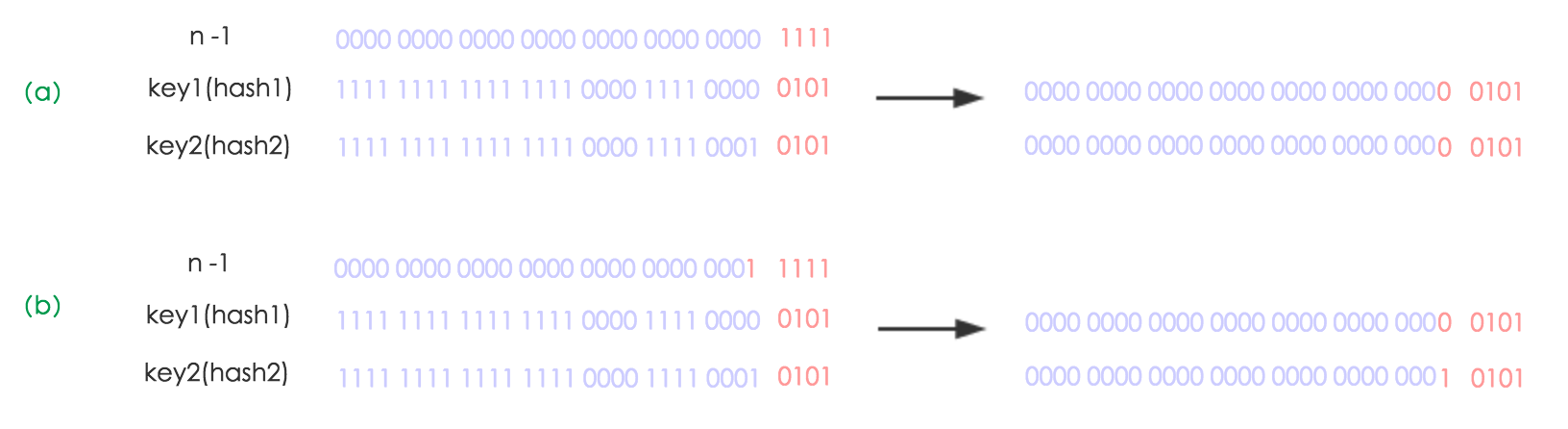``````// JDK1.8扩容过程
final Node<K,V>[] resize() {
Node<K,V>[] oldTab = table;
int oldCap = (oldTab == null) ? 0 : oldTab.length; // 原容量
int oldThr = threshold; // 原阈值
int newCap, newThr = 0; // 新容量、新阈值
if (oldCap > 0) {
// 容量已超过最大值就不再扩充了，就只好随你碰撞去吧
if (oldCap >= MAXIMUM_CAPACITY) {
threshold = Integer.MAX_VALUE;
return oldTab;
}
// 没超过最大值，就扩充为原来的2倍
else if ((newCap = oldCap << 1) < MAXIMUM_CAPACITY &&
oldCap >= DEFAULT_INITIAL_CAPACITY)
newThr = oldThr << 1; // double threshold
}
// oldThr变量保存着初始容量
else if (oldThr > 0) // initial capacity was placed in threshold
newCap = oldThr;
else {               // zero initial threshold signifies using defaults
// oldThr、oldCap都为null，表示使用默认值
newCap = DEFAULT_INITIAL_CAPACITY;
}
// 计算新的阈值
if (newThr == 0) {
float ft = (float)newCap * loadFactor;
newThr = (newCap < MAXIMUM_CAPACITY && ft < (float)MAXIMUM_CAPACITY ?
(int)ft : Integer.MAX_VALUE);
}
threshold = newThr;
@SuppressWarnings({"rawtypes"，"unchecked"})
Node<K,V>[] newTab = (Node<K,V>[])new Node[newCap]; // 创建新table
table = newTab;
if (oldTab != null) {
// 把每个bucket中的节点都移动到新的table中
for (int j = 0; j < oldCap; ++j) {
Node<K,V> e;
if ((e = oldTab[j]) != null) {
oldTab[j] = null;
if (e.next == null) // 当前bucket只有一个节点
newTab[e.hash & (newCap - 1)] = e;
else if (e instanceof TreeNode) // 当前bucket已经是红黑树结构，直接操作红黑树
((TreeNode<K,V>)e).split(this, newTab, j, oldCap);
else { // 普通链表，则将该链表分为原索引，原索引+oldCap两个位置的两条链表
Node<K,V> loHead = null, loTail = null;
Node<K,V> hiHead = null, hiTail = null;
Node<K,V> next;
do {
next = e.next;
// 原索引
if ((e.hash & oldCap) == 0) {
if (loTail == null)
else
loTail.next = e;
loTail = e;
}
// 原索引+oldCap
else {
if (hiTail == null)
else
hiTail.next = e;
hiTail = e;
}
} while ((e = next) != null);
// 原索引放到bucket里，节点相对位置不变
if (loTail != null) {
loTail.next = null;
}
// 原索引+oldCap放到bucket里，节点相对位置不变
if (hiTail != null) {
hiTail.next = null;
}
}
}
}
}
return newTab;
}
``````

### 三、HashMap在JDK8与JDK7中实现的不同点

• 存储结构：JDK8中使用了数组+链表+红黑树的形式，JDK7中为数组+链表，无红黑树；
• put方法：JDK8添加新元素是添加到链表尾部，JDK7是头插法；
• resize：
• JDK7：需要重新计算元素在新数组中的位置，并且原来在同一数组索引中的元素，若扩容后仍然在同一索引处，相对位置会颠倒(头插法)；
• JDK8：不需要重新计算元素在新数组中的索引，并且原来在同一数组索引中的元素，若扩容后仍然在同一数组索引处，相对位置不变。

### 四、为什么JDK8中链表转为红黑树的阈值是8

TreeNodes占用空间是普通Nodes的两倍，所以只有当bin包含足够多的节点时才会转成TreeNodes，而是否足够多就是由TREEIFY_THRESHOLD的值决定的。当bin中节点数变少时，又会转成普通的bin。并且我们查看源码的时候发现，链表长度达到8就转成红黑树，当长度降到6就转成普通bin。

``````Because TreeNodes are about twice the size of regular nodes, we
use them only when bins contain enough nodes to warrant use
(see TREEIFY_THRESHOLD). And when they become too small (due to
removal or resizing) they are converted back to plain bins.  In
usages with well-distributed user hashCodes, tree bins are
rarely used.  Ideally, under random hashCodes, the frequency of
nodes in bins follows a Poisson distribution
(http://en.wikipedia.org/wiki/Poisson_distribution) with a
parameter of about 0.5 on average for the default resizing
threshold of 0.75, although with a large variance because of
resizing granularity. Ignoring variance, the expected
occurrences of list size k are (exp(-0.5) * pow(0.5, k) /
factorial(k)). The first values are:
0:    0.60653066
1:    0.30326533
2:    0.07581633
3:    0.01263606
4:    0.00157952
5:    0.00015795
6:    0.00001316
7:    0.00000094
8:    0.00000006
more: less than 1 in ten million
``````

### 五、HashMap的死循环问题

``````void transfer(Entry[] newTable, boolean rehash) {
int newCapacity = newTable.length;
for (Entry<K,V> e : table) {
while(null != e) {
Entry<K,V> next = e.next;
if (rehash) {
e.hash = null == e.key ? 0 : hash(e.key);
}
int i = indexFor(e.hash, newCapacity);
e.next = newTable[i];
newTable[i] = e;
e = next;
}
}
}
``````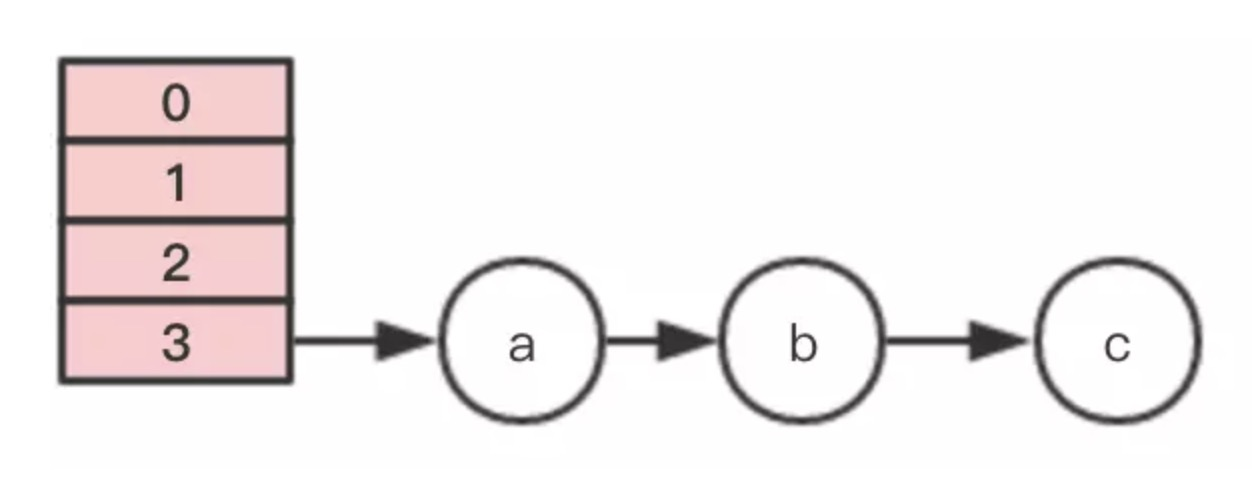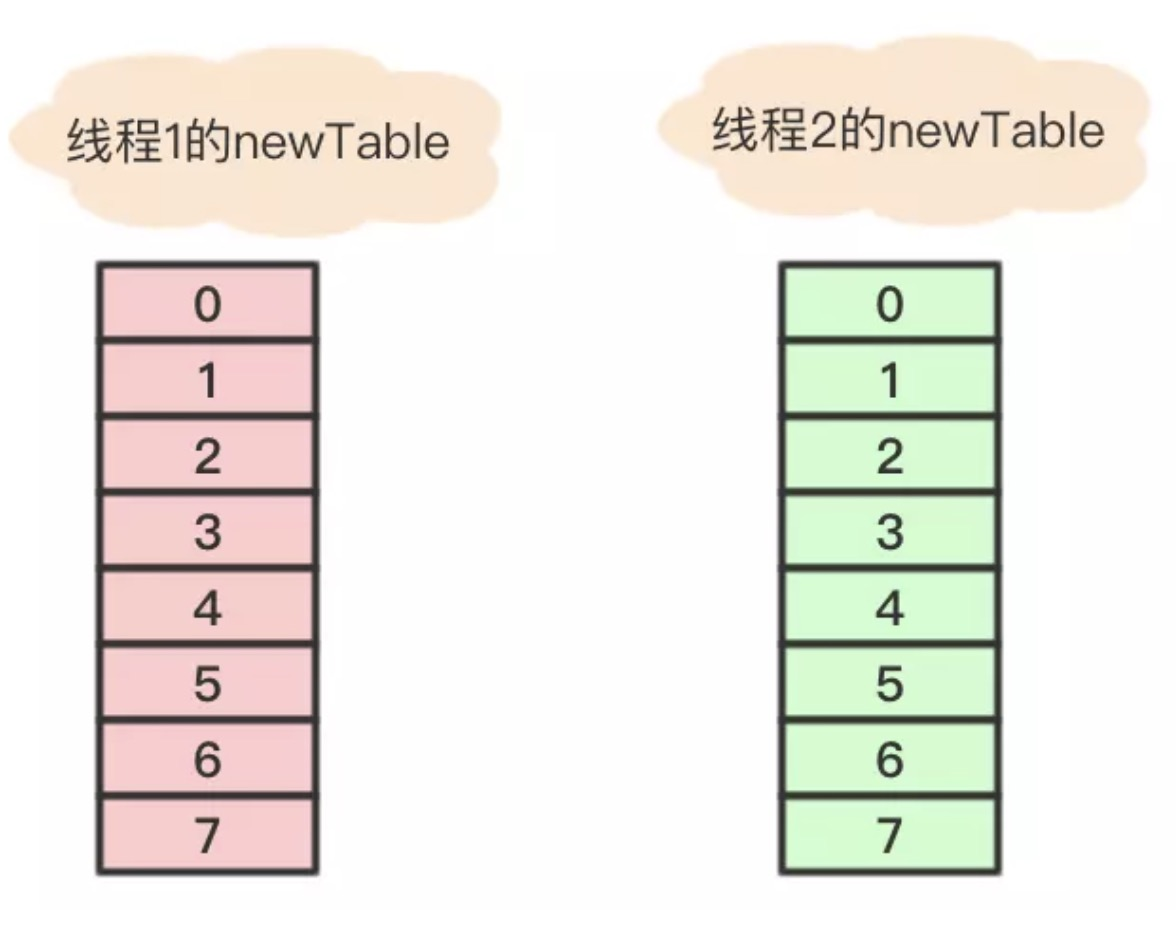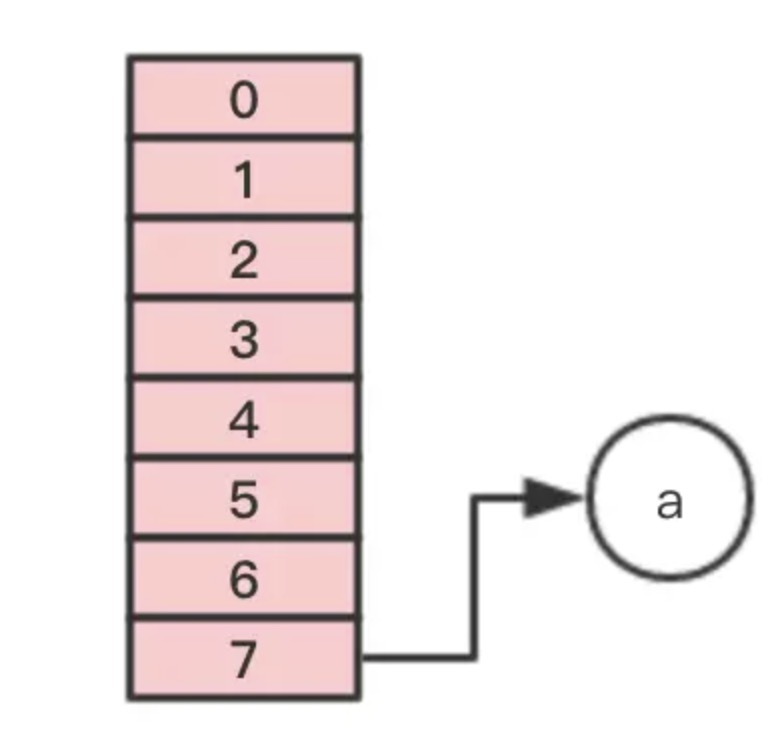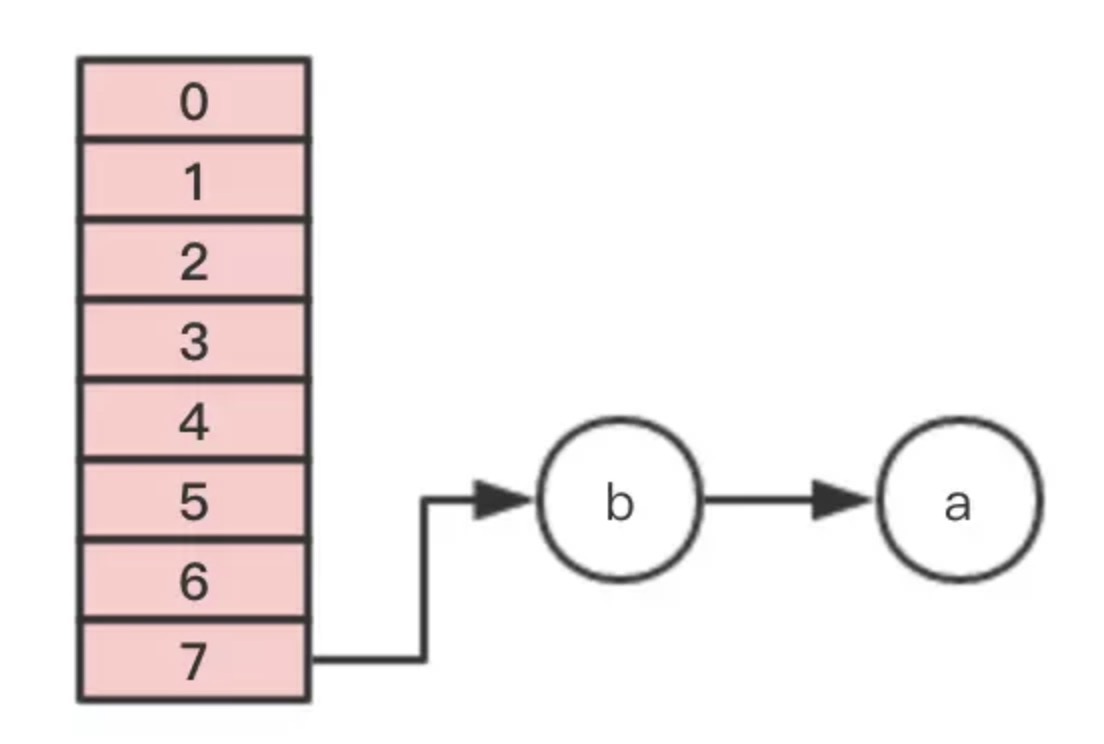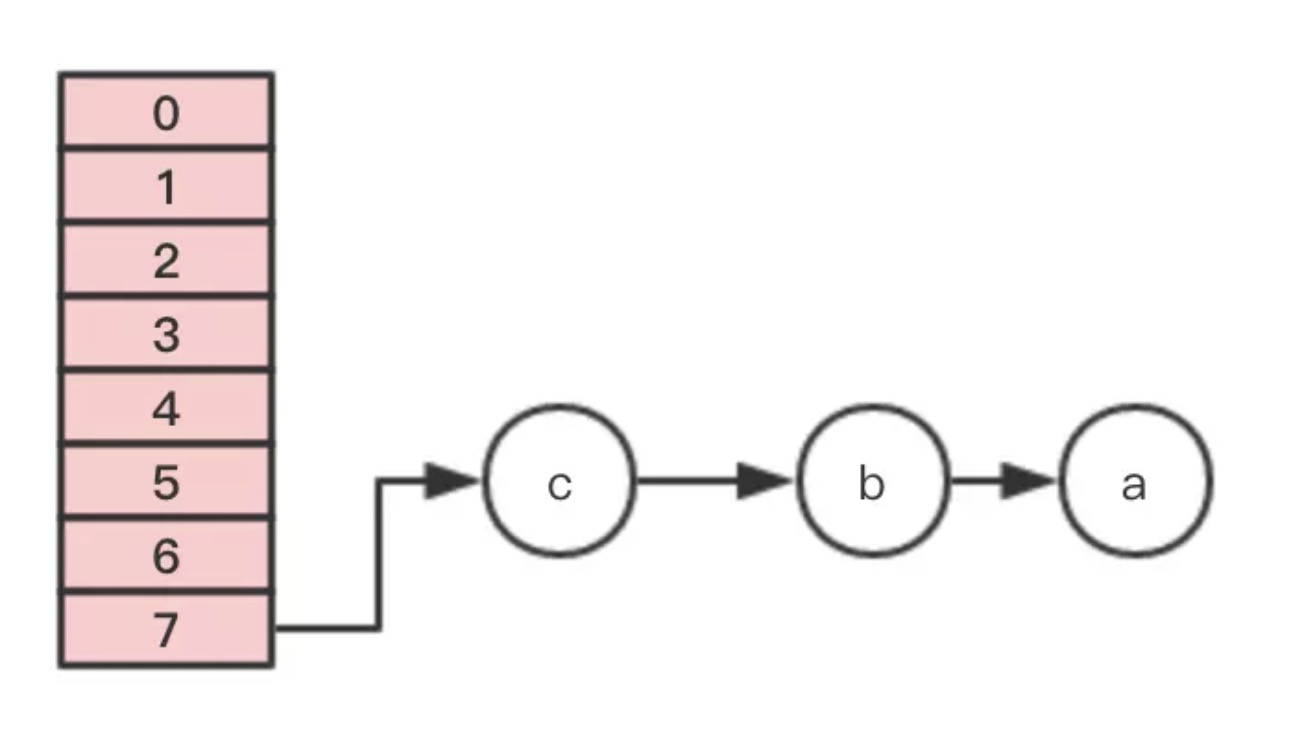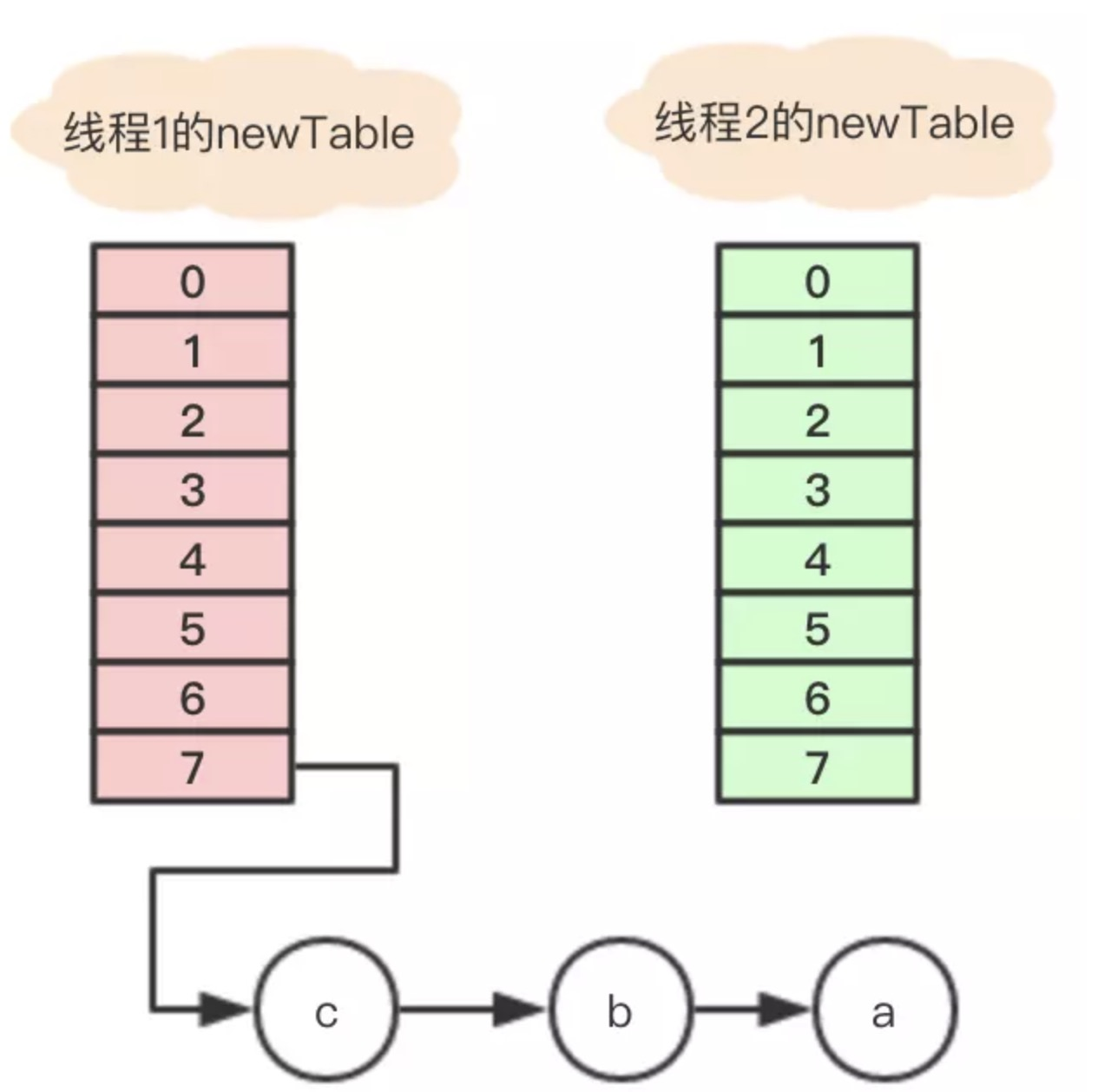``````Entry<K,V> next = e.next;
int i = indexFor(e.hash, newCapacity);
e.next = newTable[i];
newTable[i] = e;
e = next;
``````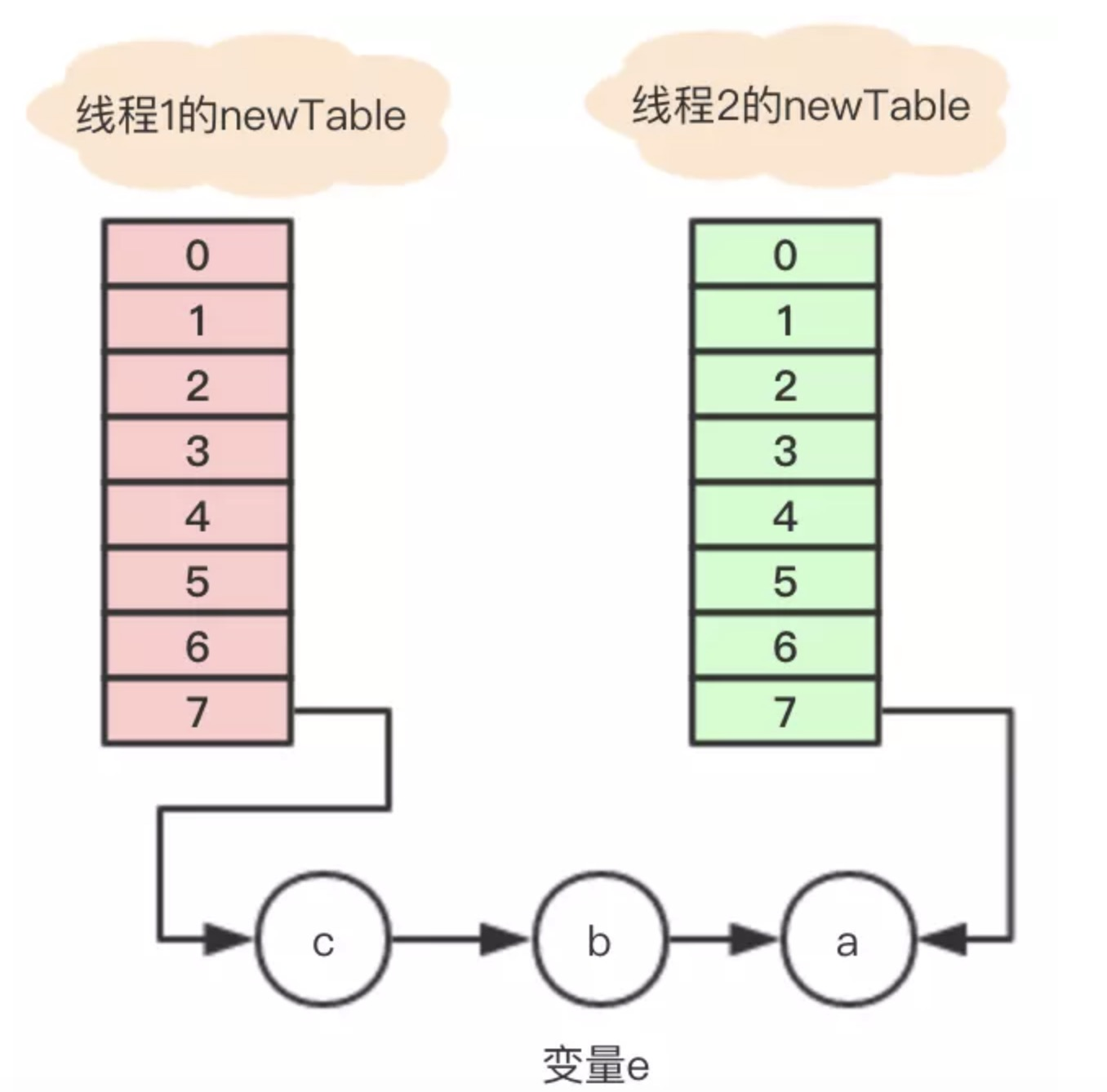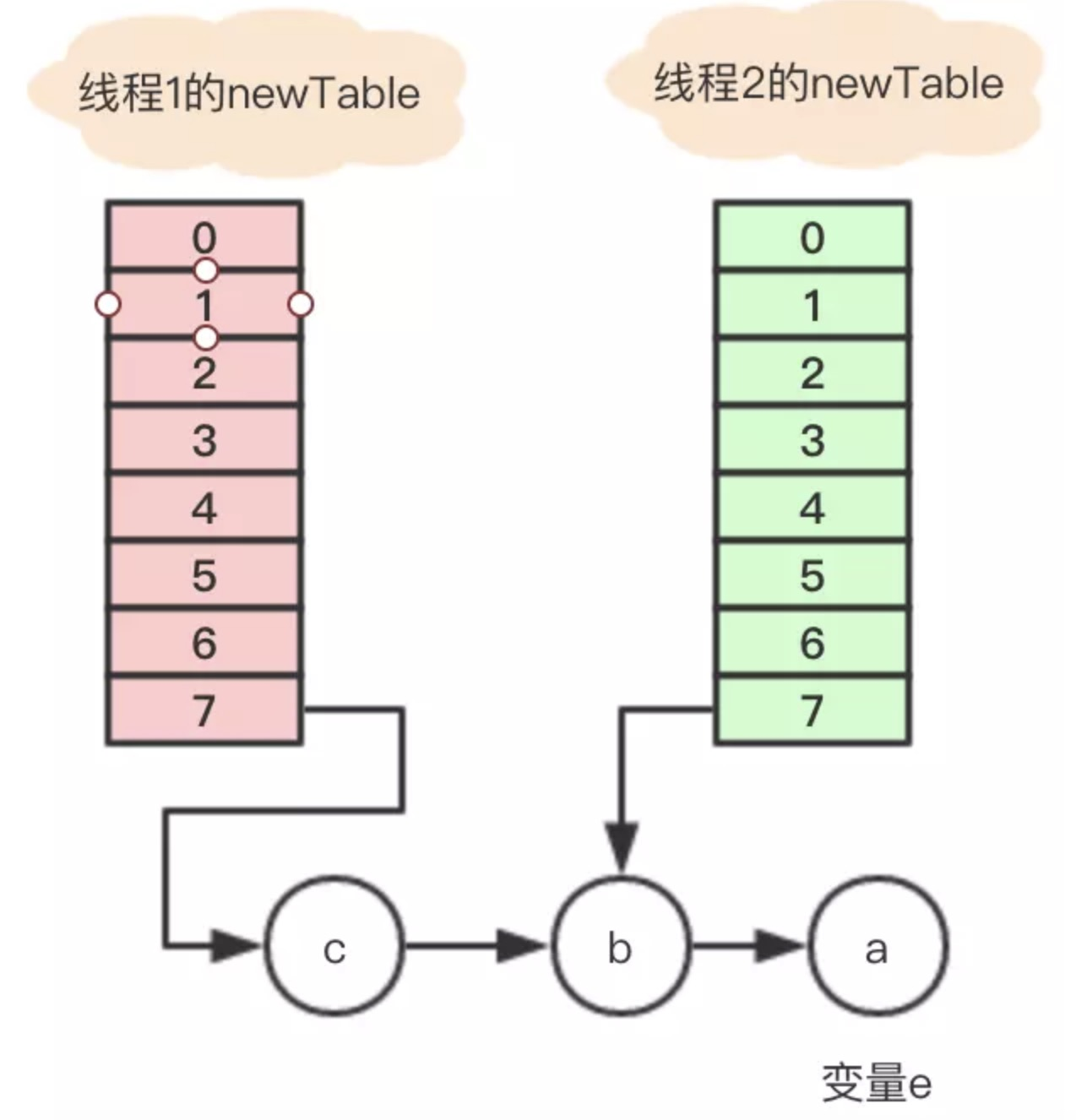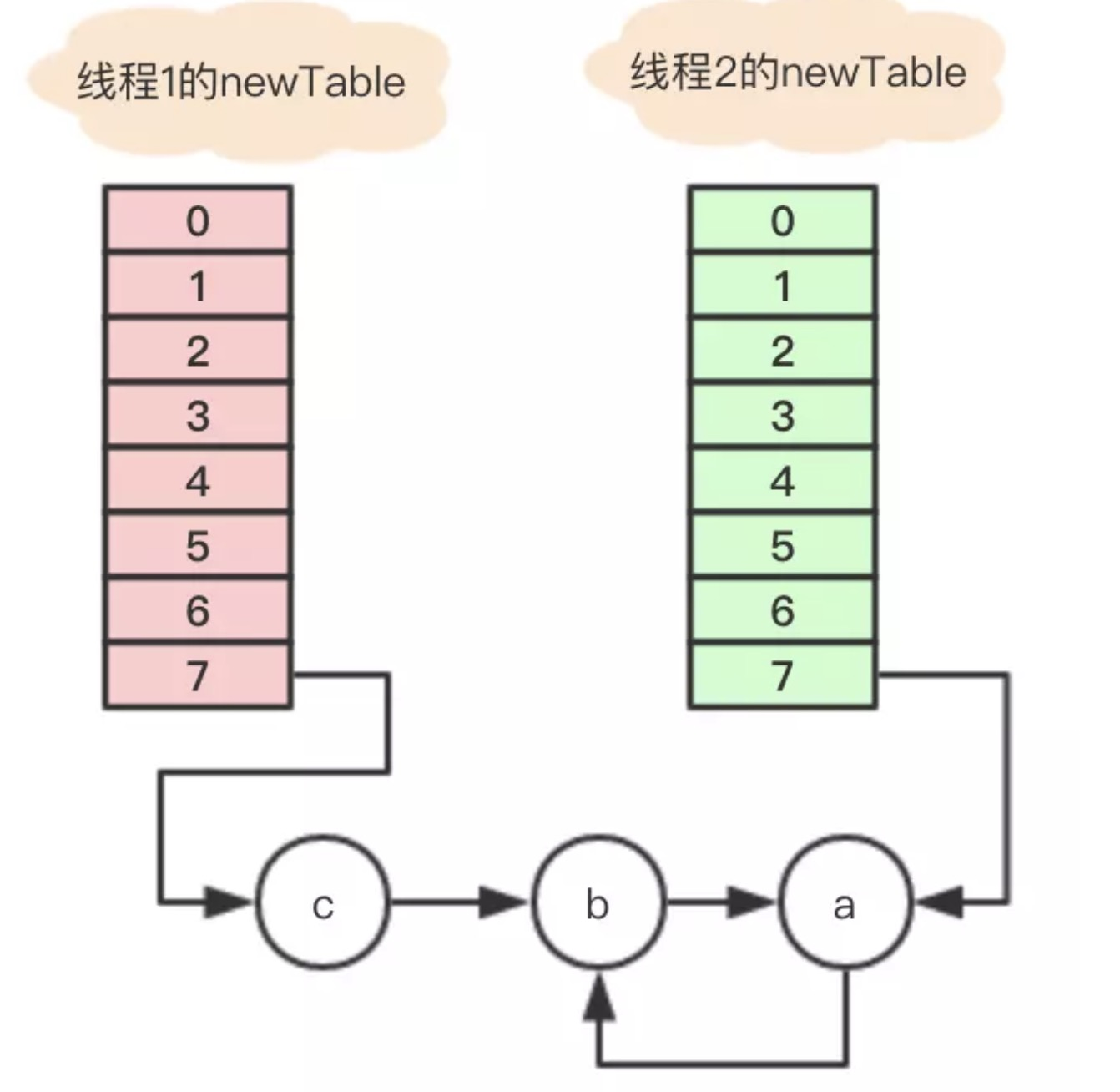### 参考文章